### Home > MC1 > Chapter 9 > Lesson 9.2.5 > Problem9-104

9-104.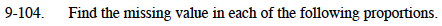For help solving proportions, refer to the Math Notes box below.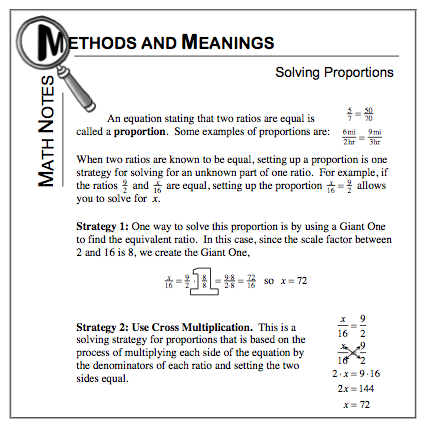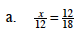We can choose cross multiplication, which is Strategy 2 in the Math Notes box above.
Your expression would then look something like this: 18x = (12)(12) Can you find the value of x now?

How many 18's are in 144? You can figure this out by dividing 144 by 18.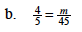$\text{Here, try using a Giant One to change }\frac{4}{5} \text{ to a fraction over 45. This will give you the value of }m.$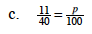Which strategy do you like to use more? Both will work here, so remember to show your work!

The value of p is 27.5.